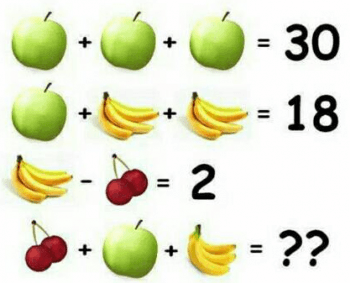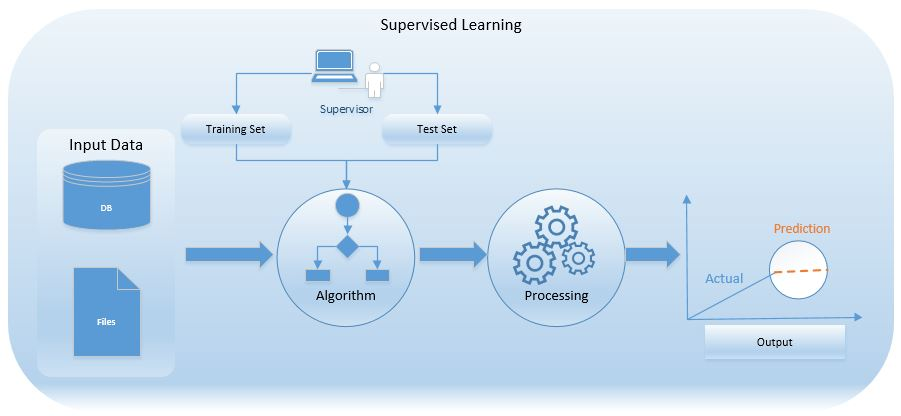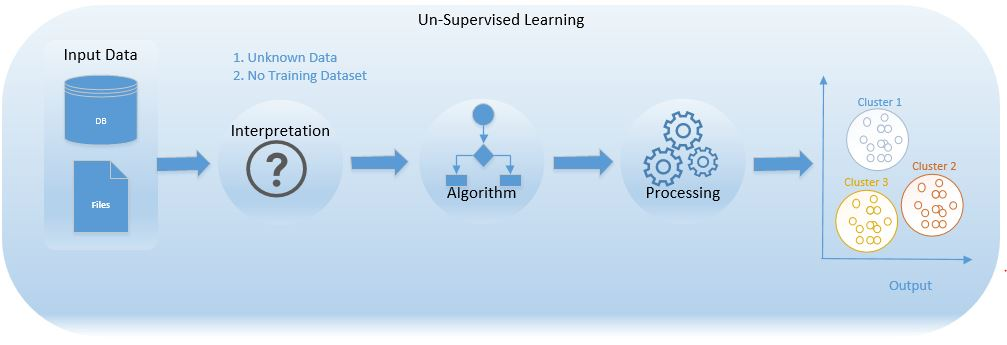# What is Machine Learning?

What is Machine Learning?

Some of you might be smiling and saying – why this article now? Since we have already published couple of articles on Regression and Recommendation Engine. But then we also got some of the queries saying – what is Machine Learning? Where do we use it? How is it different from normal Computer programming or Computation?

So we thought of putting up this article and address some of the queries above. We are trying our best to give as less technical as possible and more pictorial.

Till today, we have been defining Rules first and then making decisions, like – we define the rules for giving loan to customer. E.g. Age should be between 18 & 50, Income should be above 10000, etc. Then based on those rules lending was done. So who qualify those rules? Still there are delinquents (defaulters).

But using Machine Learning Algorithms – we will take historical data of all the loans given and then decide who should be given loan and who shouldn’t be.

Below image is best and simple example of machine learning. Machines/Computer is Trained with first three examples and asked last one as question. Computer can answer Fourth one.From first three rows are Training Data and it makes prediction on fourth row. Although this is just one part of Machine Learning.

There are two types of Machine Learning Algorithms – Supervised Learning (Learn from historical data and predict for new data) and Unsupervised Learning (e.g. Grouping similar characteristics elements).

## Supervised Learning

Model is trained on labelled data set or model is able to predict with help of labelled data set.

We show output to a model, we have given data and we know what output is. Here we show output to a model and we tell it to learn based on output shown. We show model output, gives inputs and it learns itself.

We teach model, then with that knowledge have to predict future instance.

What is labelled data set?

Data for which you know the already target answers is called labelled data set

Types

1. Classification: when output variable is categorical like (yes/no, true/false) E.g.:- Email is spam or not? Here we need to teach machine/Model what is spam mail looks like (done by using spam filters, identifying keywords like lottery, prices etc.)
2. Regression: Relationship between two or more variables where change in one variable is associated with change in other variable. E.g.:- Temperature & Humidity, where Temperature is Independent variable & Humidity is Dependent variable, As Temperature increases, Humidity decreases so they both are correlated (inversely Proportional), we are training model that one variable is dependent on the other. So equation is formed to predict future values.

Real world examples

1. Bio-metric attendance systems where you train the machine after couple of inputs (of your bio-metric identity, be it thumb or iris or ear-lobe, etc.), machine can validate your future input and identify you.
2. Cortana or any speech automated system in your mobile phone trains your voice and then starts working based on this training.

What Statistical Algorithms are used – Decision Tree, Logistic regression, Support vector machine## Unsupervised Learning

Models are Trained using data that is unlabelled, here machine tries to identify patterns and gives output.

We don’t teach model even we don’t show output to a model but model learns based on its own capability, it learns what should be the input & what should be the output.

What is unlabelled data set?

We don’t have a data set with right answers (we have data without labels)

Types

1. Clustering – Method of dividing objects into clusters which are similar between them and are dissimilar to the objects belonging to another cluster. E.g. k Means and Hierarchy Clustering.
2. Association – Discovering the probability of co-occurrence of Items in a collection. This algorithm is also known as Market Basket Analysis. E.g. typically we see on eCommerce portals, where it shows Customers “who bought this” “Also Bought”.

What Statistical Algorithms are used – K Means Clustering, Hierarchical ClusteringAll these Statistical Algorithms are part of “Classical Machine Learning”.  So other Statistical Algorithms are – Reinforcement Learning, Ensembles (this is used for calculating CIBIL/FICO scores) and Neural Networks and Deep Learning.